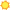# Related Occupations for Task15-2041.00 - Statisticians15-2041.01 BiostatisticiansBright Outlook   2 closely related tasks Apply research or simulation results to extend biological theory or recommend new research projects. Develop or use mathematical models to track changes in biological phenomena, such as the spread of infectious diseases. 15-2031.00 Operations Research Analysts2 closely related tasks Define data requirements and gather and validate information, applying judgment and statistical tests. Break systems into their components, assign numerical values to each component, and examine the mathematical relationships between them. 15-2021.00 MathematiciansApply mathematical theories and techniques to the solution of practical problems in business, engineering, the sciences, or other fields. 15-2091.00 Mathematical Technicians Apply standardized mathematical formulas, principles, and methodology to the solution of technological problems involving engineering or physical science. 15-1132.00 Software Developers, ApplicationsDesign, develop and modify software systems, using scientific analysis and mathematical models to predict and measure outcome and consequences of design.